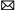# Motor QUIZ

1. A rotating magnetic field is the key to the operation of AC motors. Give a brief explanation of its principle of operation.
2. Compare synchronous speed and actual speed of an AC motor.
3. Calculate the synchronous speed of a six-pole AC motor operated from a standard voltage source.
4. Why is the induction motor so named?
5. Outline the operating principle of a three-phase squirrel cage induction motor.
6. Explain what effect rotor resistance has on the operation of a squirrel cage induction motor.
7. How is the direction of rotation of a squirrel-cage motor reversed?
8. If, while a three-phase induction motor is operating, power to one phase of its squirrel cage is lost, what will happen?
9. Define the term slip as it applies to an induction motor.
10. Calculate the percent slip of an induction motor having a synchronous speed of 3,600 rpm and a rated actual speed of 3,435 rpm.
11. What effect does loading have on the power factor of an AC motor?
12. What is the typical value of the locked-rotor motor current?
13. How is the speed of an induction motor determined?
14. Explain the difference between multispeed consequent pole and separate winding induction motors.
15. A wound-rotor induction motor is normally started with full external resistance in the rotor circuit that is gradually reduced to zero. How does this affect starting torque and current?
16. How is the direction of rotation of a wound-rotor induction motor changed?
17. When a wound-rotor motor is used as an adjustable speed drive, rather than only for starting purposes, what must the duty cycle of the rotor resistors be rated for?
18. State two advantages of using three-phase synchro nous motor drives in an industrial plant.

Another group:
1. What is the major difference between the starting requirements for a three-phase and a single phase induction motor?
2. a. Outline the starting sequence for a split phase induction motor.
b. How is its direction of rotation reversed?
3. Dual-voltage split-phase motors have leads that allow external connection for different line voltages. How are the start and run windings connected for high and low line voltages?
4. What is the main advantage of capacitor motors over split-phase types?
5. Name the three types of capacitor motor designs.
6. Explain how the shaded-pole motor is started.
7. What type of DC motor is constructed like a universal motor?
<- - Make a Comment - ->
You may like:Servo Motor & Drivesbuy@servomotor.cotech@servomotor.co

Category
Featured